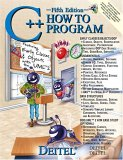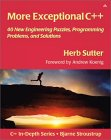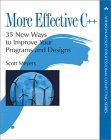# C / C++ and Dynamic memory allocation

This C / C++ tutorial covers dynamic memory allocation, memory allocation (on heap not stack), exception handling and some examples. Examples are included of "C" malloc() and free() as well as "C++" new and delete.

Program memory: Heap vs Stack

Dynamic memory is allocated on the "heap". The heap is the region of computer memory which is managed by the programmer using pointers to access the memory.

Heap memory details:
• can be accessed globally
• your limit is the physical memory limit of your system (real and virtual)
• user managed memory. Programmer must allocate and free memory.
• memory access is via pointer and thus requires dereferencing to access, requiring extra operations and time
• memory can become defragmented as memory is allocated and freed
• memory can be resized (eg using realloc())
The "stack" is the region of memory which stores declared variables in main() and all called functions. When a function returns, the memory space for the declared variables is released ("popped"). The stack is FILO (first in - lst out) and unwinds variables in memory as functions return.
• the "stack" grows and shrinks as functions push and pop local variables
• the "stack" is managed by the OS
• the default "stack" size is limited by the OS. A stack overflow occurs when this limit is exceeded (eg endless function recursion).
Example of behavior of objects declared on the heap vs stack:
```#include <iostream>
using namespace std;

class AAA
{
public:
AAA();
AAA(int);
~AAA();
private:
int ii;
};

AAA::AAA()
: ii(1)
{
cout << "Constructor called. Value of ii=" << ii << endl;
}

AAA::AAA(int _ii)
: ii(_ii)
{
cout << "Constructor called. Value of ii=" << ii << endl;
}

AAA::~AAA()
{
cout << "Destructor called. Value of ii=" << ii << endl;
}

main()
{
cout << "Start of program" << endl;
AAA a1;               // Declared on the stack.
AAA a2(2);            // Declared on the stack.
AAA *a3 = new AAA(3); // Dynamically allocated memory on the heap
AAA *a4 = new AAA(4); // Dynamically allocated memory on the heap
delete a3;            // If not called, then destructor not called
cout << "End of program" << endl;
}
```
Compile: g++ test.cpp
Run: ./a.out
```Start of program
Constructor called. Value of ii=1
Constructor called. Value of ii=2
Constructor called. Value of ii=3
Constructor called. Value of ii=4
Destructor called. Value of ii=3
End of program
Destructor called. Value of ii=2
Destructor called. Value of ii=1
```

Note:
• Stack: The objects a1 and a2 are declared on the stack. The constructors are called automatically where they are declared. The destructors are called automatically when they go out of scope (as defined by the last "}").
• Heap: Objects allocated dynamically on the heap with "new" automatically have their constructors called. Note that the destructor for a3 is called when the memory is freed with "delete". Delete is not called for a4 and thus the destructor is not called.

C malloc and free vs C++ new and delete:

C malloc() and free() do not call constructors or destructors. C++ new and delete operators are "class aware" and will call class constructors when a class is allocated with new and a destructor when delete is called. [example]

Mixing malloc with delete or new with free is undefined. In addition, C++ new and delete operators were introduced with the C++ practice of allocating memory in constructors and deallocating the same memory with a destructor.

• new/delete invokes constructor/destructor. Malloc/free will not.
• new does not need typcasting. Malloc requires typcasting the returned pointer.
• new/delete operators can be overloaded, malloc/free can not.
• new does not require you to explicitly calculate the quantity of memory required. (Unlike malloc)

C dynamic memory allocation:

Use "malloc", "calloc" and "free": File: MallocTest.cpp

```#include <stdio.h>
#include <string.h>
#include <stdlib.h>
#include <error.h>
#include <errno.h>

typedef struct
{
int ii;
double dd;
} SSS;

int main()
{
int kk, jj;

char *str1 = (char *) "This is a text string";
char *str2 = (char *) malloc(strlen(str1));

errno = 0;
SSS *s1 = (SSS *)calloc(4, sizeof(SSS));  // Memory for 4 of struct SSS
if(s1 == NULL)
{
if(errno == ENOMEM) printf("Error ENOMEM: Insufficient memory available\n");
}

strcpy(str2,str1); /* Make a copy of the string */

for(kk=0; kk < 5; kk++)
{
s1[kk].ii=kk;
}

for(jj=0; jj < 5; jj++)
{
printf("Value stored: %d\n",s1[jj].ii);
}

free(s1);
free(str2);
}
```

Compile: gcc -o MallocTest MallocTest.cpp

Run: MallocTest

```Value stored: 0
Value stored: 1
Value stored: 2
Value stored: 3
Value stored: 4
```

Manual pages:

• malloc: a memory allocator
• calloc: a memory allocator
• realloc: memory reallocator
• free: frees the memory space pointed to by ptr
• errno: obtains error number of last error
• perror: obtains error number of last error
Note that calloc() zeros memory while malloc() does not.

C++ dynamic memory allocation:

Use "new" and "delete": File: AllocNewTest.cpp

```#include <iostream>

class CCC
{
public:
CCC(){};
CCC(int);
CCC(int, double);
int ii;
double dd;
};

CCC::CCC(int _ii)
: ii(_ii)
{
};

CCC::CCC(int _ii, double _dd)
: ii(_ii), dd(_dd)
{
};

using namespace std;

main()
{
CCC *cc1 = new CCC(4, 5.5);   // Pointer. Contructor called.
CCC *cc2 = new CCC;        // Pointer to an array of objects.
CCC &cc3 = *new CCC;          // Reference
CCC **c4 = new CCC * ;     // Array of pointers to pointers

cc1->ii   = 5;
cc2.ii = 6;
cc3.ii    = 7;
c4     = new CCC(8);
c4     = new CCC(9);

cout << cc1->ii   << endl;
cout << cc2.ii << endl;
cout << cc3.ii    << endl;
cout << c4->ii << endl;
cout << c4->ii << endl;

delete cc1;
delete [] cc2;
delete & cc3;

delete [] c4;   // First delete pointer content
delete [] c4;
delete [] c4;      // then delete array of pointers
}
```

Note the difference between:
• new CCC(3) creates memory for a single object who's integer member is set to 3.
• new CCC creates memory for three objects of type CCC and no variables are set.

Compile: g++ -o AllocNewTest AllocNewTest.cpp

Run: ./a.out
```5
6
7
8
9
```

C function returning a pointer vs C++ function returning a copy:

C function returning a pointer:
```#include <stdio.h>
#include <stdlib.h>

char *fnConvert(int _ii)
{
char *str = (char *) malloc(10);  /* Return 10 character string */
if(str == NULL)
{
fprintf(stderr,"Error: Memory allocation failed.\n");
perror("malloc");
}
sprintf(str, "%d", _ii);
return str;
}

main()
{
char *s1=fnConvert( 34567 );

printf("%s\n", s1);

free(s1);
}
```

C++ function returning a copy:

```#include <iostream>
#include <sstream>
#include <string>

using namespace std;

string fnConvert(int _ii)
{
ostringstream ost;
ost << _ii;
return ost.str();
}

main()
{
cout << fnConvert( 34567 ) << endl;
}
```

Note: The STL string class copy constructor is employed to return a copy (return by value). Yes the variable "ost" is out of scope once we leave the function, but the copy of its contents is valid. Do not "return ost.str().c_str()" as this pointer is out of scope once the procesing leaves the function and the data lost.

C++ dynamic memory allocation exception handling:

Use exception handling:

```#include <iostream>

using namespace std;

main()
{
int ii;
double *ptr;

try
{
for( ii=0; ii < 5000000; ii++)
{
ptr[ii] = new double;
}
}

{
cout << "Error on loop number: " << ii << endl;
cout << "Memory allocation exception occurred: "
<< memmoryAllocationException.what()
<< endl;
}
catch(...)
}
cout << "Unrecognized exception" << endl;
{
}
```

Compile: g++ -o AllocNewTest AllocNewTest.cpp

Run:

• Observer system limits: ulimit -a
• Set system limits: ulimit -m 100
• Run with fewer privileges: nice -n 19 AllocNewTest

C++ Virtual Destructors:

Using polymorphism to delete dynamically allocated objects by their base class pointer: Declare base class destructor virtual so that the delete operator can be applied to the base class pointer. The derived class destructor is called first, before the base class destructor.

```#include <iostream>
using namespace std;

class Base
{
public:
Base(){};
virtual ~Base(){ cout << "Base class destructor called" << endl; }
};

class Derived : public Base
{
public:
Derived(){};
~Derived(){ cout << "Derived class destructor called" << endl; }
};

main()
{
Base *ptr = new Derived();
delete ptr;
}
```

Resutls:

Derived class destructor called
Base class destructor called

Note:

• If the delete operator is applied to the base class and the destructor is NOT virtual, then this will cause a memory leak as only a portion of the memory is freed.
• Base class destructor is not pure virtual (set =0) or there would be no base class implementation of the destructor..
• Class contructors can NOT be virtual.

Notes and Tutorials:Books:C++ How to Program by Harvey M. Deitel, Paul J. Deitel ISBN #0131857576, Prentice Hall Fifth edition. The first edition of this book (and Professor Sheely at UTA) taught me to program C++. It is complete and covers all the nuances of the C++ language. It also has good code examples. Good for both learning and reference.Exceptional C++: 47 Engineering Puzzles, Programming Problems and Solutions by Herb Sutter ISBN #0201615622, Addison-Wesley Professional Advanced C++ features and STL.More Exceptional C++ by Herb Sutter ISBN #020170434X, Addison-Wesley ProfessionalEffective C++: 50 Specific Ways to Improve Your Programs and Design (2nd Edition) by Scott Meyers ISBN #0201924889, Addison-Wesley ProfessionalMore Effective C++: 35 New Ways to improve your Programs and Designs by Scott Meyers ISBN #020163371X, Addison-Wesley Professional